# User:IssaRice/Linear algebra/Riesz representation theorem

Let's take the case where$V = \mathbf R^n$ and the inner product is the usual dot product. What does the Riesz representation theorem say in this case? It says that if we have a linear functional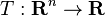$T : \mathbf R^n \to \mathbf R$ then we can write$T$ as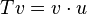$Tv = v\cdot u$ for some vector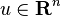$u \in \mathbf R^n$. But we already know (from the correspondence between matrices and linear transformations) that we can represent$T$ as a 1-by-n matrix. And v can be thought of as a n-by-1 matrix. And now the dot product is the same thing as the matrix multiplication!
If$\sigma = (e_1, \ldots, e_n)$ is the standard basis of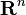$\mathbf R^n$ and$(1)$ is the standard basis of$\mathbf R$, then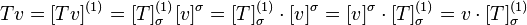$Tv = [Tv]^{(1)} = [T]_\sigma^{(1)} [v]^\sigma = [T]_\sigma^{(1)} \cdot [v]^\sigma = [v]^\sigma \cdot [T]_\sigma^{(1)} = v \cdot [T]_\sigma^{(1)}$.
So in the case$V = \mathbf R^n$ we can understand the Riesz representation theorem as saying something we already knew. What Riesz representation theorem does is extend this same sort of "representability" to all finite-dimensional inner product spaces V and all linear functionals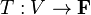$T : V \to \mathbf F$.
this video talks about this for$\mathbf R^2$